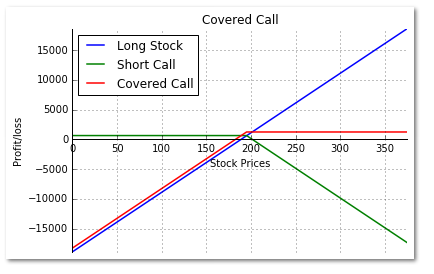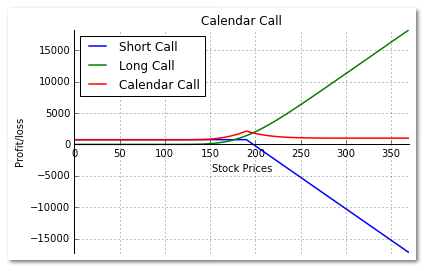# Write Covered Call Strategy In Python

##### Introduction

Traders in the derivative market often exercise one of the following: Call Option or Put Option.

“Call Option” is a financial contract between a buyer and seller, whereby the buyer has the right, but not the obligation, to buy an agreed quantity of a financial instrument from the seller of the option at a certain time for a certain price (the strike price). The “Put Option” serves the opposite.

In a “Covered Call”, the seller of the call options owns the corresponding amount of the underlying instrument.

A Covered Call is an income generating option strategy which involves two legs:

• Selling an Out of the money (OTM) call option

If the call is sold simultaneously along with the stock purchase, the strategy is referred to as a “buy-write” strategy.

In a Covered Call, the trader holds a neutral to a bullish outlook. Covered Call is a net debit transaction because you pay for the stock and receive a small premium for the call option sold.

The idea of this blog post is to elaborate on the covered call strategy by an example and to plot its payoff using Python. The post also highlights "Calendar Call" as it is a modification of the Covered Call strategy.

Let us take an example straight away to understand the working of a Covered Call, its payoff, and the risk involved in the strategy.

#### Covered Call Strategy example

SBI stock is trading at Rs. 189 on April 29th, 2015. Leg 1: Buy 100 shares of the stock for Rs. 189 Leg 2: Sell the 195 strike May 26th, 2015 Call for Rs.6.30, Lot size – 100 shares

The amount paid to take these two positions equals - the Stock price paid minus call premium received, i.e. Rs. 18,900 (Stock purchase) – Rs. 630 (Premium received) = Rs. 18,270

If the stock price rises above the call strike of 195, it will be exercised, and the stock will be sold. However, the strategy will make a profit because you are covered by the stock you own.

Say, the stock price at expiration is Rs. 200. The profit made is given by:

 Profit = Call premium received + ((Call strike - stock price paid) x Shares)
Profit = Rs. 630 + ((195 – 189) x 100) = Rs. 1,230

If the stock falls below the initial stock purchase price, your long position is at a loss, but you have some cushion from the call premium received by selling the call.

Say, the stock falls and is at Rs. 180 on expiration. The loss incurred is given by

Loss = Call premium received + ((stock price at expiration - stock price paid) x Shares)
Loss = Rs. 630 + ((180 – 189) x 100) = - Rs. 270

One can employ the Covered Call strategy by selling calls on a monthly basis and thus generating monthly income by way of premiums.

The Risk-Reward Profile for Covered Call is as given below:

2. Maximum Reward – (Call strike - the stock price paid) + call premium received

#### Python code for Covered Call Payoff chart

Below is the code for Long Stock, Short Call and Covered call payoff chart in Python.

# Covered Call

import numpy as np
import matplotlib.pyplot as plt

s0=189 # Initial stock price
k=195;c=6.30; # Strike price and Premium of the option
shares = 100 # Shares per lot
sT = np.arange(0,2*s0,5) # Stock Price at expiration of the Call# Profit/loss from long stock position
y1= (sT-s0) * shares# Payoff from a Short Call Option
y2 = np.where(sT > k,((k - sT) + c) * shares, c * shares)# Payoff from a Covered Call
y3 = np.where(sT > k,((k - s0) + c) * shares,((sT- s0) + c) * shares )# Create a plot using matplotlib
fig, ax = plt.subplots()
ax.spines['top'].set_visible(False) # Top border removed
ax.spines['right'].set_visible(False) # Right border removed
ax.spines['bottom'].set_position('zero') # Sets the X-axis in the center
ax.tick_params(top=False, right=False) # Removes the tick-marks on the RHS

plt.plot(sT,y1,lw=1.5,label='Long Stock')
plt.plot(sT,y2,lw=1.5,label='Short Call')
plt.plot(sT,y3,lw=1.5,label='Covered Call')

plt.title('Covered Call')
plt.xlabel('Stock Prices')
plt.ylabel('Profit/loss')

plt.grid(True)
plt.axis('tight')
plt.legend(loc=0)
plt.show()

We use the matplotlib library to plot the charts. We first create an empty figure and add a subplot to it. Then we remove the top & the right border and move the X-axis at the centre. Using the plt.plot function we plot payoffs for Long stock, Short Call, and the Covered Call. Finally, we add the title, labels, and the legend in the chart.### Calendar Call Strategy

Calendar Call is a variation of a Covered Call strategy, where the long stock position is substituted with a long-term long call option instead.

The Calendar Call strategy involves two legs:

• Buying a long-term expiration call with a near the money strike price and
• Selling a short-term call option with the same strike price

With a Calendar Call, the outlook of the trader is neutral to bullish. This is a net debit transaction because calls bought will be more expensive than the calls sold, which have less time value. The Calendar Call like the Covered Call can be an income generating strategy by way of selling short-term calls on a monthly basis.

Let us take an example of a Calendar Call to understand its payoff, and the risk involved in the strategy.

#### Example:

XYZ stock is trading at Rs. 187 on May 2nd, 2015, with historical volatility at 40% and a risk-free rate of 8%.

Leg 1: Buy the 190 strike July 28th, 2015 Call for Rs.20.70, Lot size – 100 shares

Leg 2: Sell the 190 strike May 26th, 2015 Call for Rs.7.50, Lot size – 100 shares

##### 1st Scenario:

At May expiration the stock falls to Rs.180

The long call is worth approximately Rs.8.80; loss of Rs.11.90

Short calls expire worthlessly, and we pocket the profit of Rs.7.50

Total position – loss of Rs.4.40

##### 2nd Scenario:

At May expiration the stock rises to Rs.205

In this case, the stock has risen above the strike price. The short call will get exercised. The price of the long call is approximately Rs.23.60; profit of 2.90.

Sell long call for a profit of Rs.2.90

Keep short call premium of Rs.7.50

Short call expires at Rs.15 ITM. Buy stock at Rs.205; Sell stock at Rs.190 – loss of Rs.15

Hence total position – loss of Rs.4.60

#### The Risk-Reward Profile for Calendar Call is as given below:

• Maximum Risk - Limited to the net debit paid
• Maximum Reward – (Value of the long call at the time of the short call expiration, when the stock price is at the strike price) minus (net debit)

### Python code for Calendar Call Payoff chart

Below is the code for Short Call, Long Call Premium, and the Calendar call payoff.

# Calendar Call Strategy

import p4f
import numpy as np
from datetime import datetime
import matplotlib.pyplot as plt

s0 = 187 # Initial stock price on May 2nd, 2015
k = 190 # Strike price of the May 26th 2015 and July 28th 2015 Call Option
cs = 7.50; # Premium of the Short call on May 2nd, 2015
cl = 20.70; # Premium of the Long call on May 2nd, 2015
shares = 100 # Shares per lot
sT = np.arange(0,2*s0,5) # Stock Price at expiration of the Call
sigma = 0.4 # Historical Volatility
r = 0.08 # Risk-free interest rate

t = datetime(2015,7,28) - datetime(2015,5,26) ; T = t.days / 365;# Payoff from the May 26th 2015 Short Call Option at Expiration
y1 = np.where(sT > k,((k - sT) + cs) * shares, cs * shares)# Value of the July 28th 2015 long Call Option on May 26th 2015
lc_value = p4f.bs_call(sT,k,T,r,sigma) * shares# Payoff from the Calendar Call
y2 = y1 + (lc_value - cl)# Create a plot using matplotlib
fig, ax = plt.subplots()
ax.spines['top'].set_visible(False) # Top border removed
ax.spines['right'].set_visible(False) # Right border removed
ax.spines['bottom'].set_position('zero') # Sets the X-axis in the center
ax.tick_params(top=False, right=False) # Removes the tick-marks on the RHS

plt.plot(sT,y1,lw=1.5,label='Short Call')
plt.plot(sT,lc_value,lw=1.5,label='Long Call')
plt.plot(sT,y2,lw=1.5,label='Calendar Call')

plt.title('Calendar Call')
plt.xlabel('Stock Prices')
plt.ylabel('Profit/loss')

plt.grid(True)
plt.axis('tight')
plt.legend(loc=0)
plt.show()We use the matplotlib library to plot the charts. We first create an empty figure and add a subplot to it. Then we remove the top & the right border and move the X-axis at the centre Using the plt.plot function we plot payoffs for Short Call, Long Call Premium, and the Calendar Call. Finally, we add the Title, labels, and the legend in the chart.

If you liked what you read, please give us feedback in the comments section below. For more such strategies and python codes, go through our blogs section. If you are looking for python codes to build technical indicators like Moving Average or Ease of Movement, you will find it here.  In case you are wondering what makes Python most preferred language for Algorithmic Traders, we have a cool infographic about it.

### Next Step

If you are a coder or a tech professional looking to start your own automated trading desk. Learn automated trading from live Interactive lectures by daily-practitioners. Executive Programme in Algorithmic Trading covers training modules like Statistics & Econometrics, Financial Computing & Technology, and Algorithmic & Quantitative Trading. Enroll now!

Disclaimer: All investments and trading in the stock market involve risk. Any decisions to place trades in the financial markets, including trading in stock or options or other financial instruments is a personal decision that should only be made after thorough research, including a personal risk and financial assessment and the engagement of professional assistance to the extent you believe necessary. The trading strategies or related information mentioned in this article is for informational purposes only.